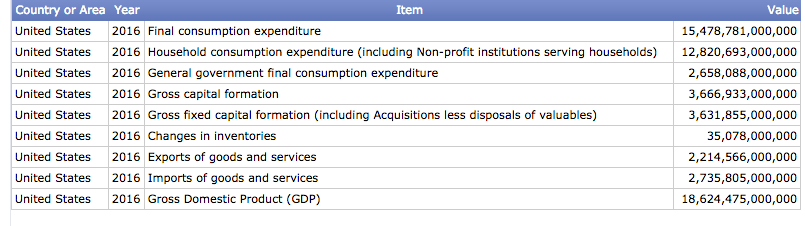Expenditure Approach

What is Expenditure Approach?

Definition: The gross domestic product (GDP) of a country can be calculated by adding total spending in the economy to total investments and net exports. This is the expenditure approach to arriving at the GDP.

What does Expenditure Approach mean?

The expenditure approach calculates GDP by adding:

⇨ Private consumption expenditure – this refers to the amount spent by households on their individual needs.

⇨ Government consumption expenditure – expenditure by the government is an important component of total expenditure. The government can increase expenditure to boost the economy.

⇨ Gross capital formation – the amount invested by the private sector as well as the government sector in capital assets is included in this category.

⇨ Exports minus imports – the net figure derived by subtracting imports from exports is added to the country’s GDP. Note that this component of GDP may be negative. This would happen if imports exceed exports.

In addition to the expenditure approach, there are two other methods for calculating a nation’s GDP. The second method, which is known as the production approach, estimates the gross value of output from each sector. Intermediate consumption is reduced from this figure to arrive at the GDP.

The third method is called the income approach. As its name implies, this method adds all forms of income (wages, profits, rents, and interest income) to calculate GDP.

Example of Expenditure Approach

The GDP of the U.S. can be calculated by using the expenditure approach. According to data provided by the United Nations Statistics Division, the different components of the U.S. gross domestic product in 2016 were:Source – United Nations

The country’s GDP of \$18.62 trillion is arrived at by adding the final consumption figure to investments and net exports.

Summary

The expenditure approach is one of three methods to calculate GDP. This method and the other two, which are the called the production approach and the income approach, should result in the same GDP.

Get the latest CPA news and discounts!

=Get My 4 Resources I Used to Pass the CPA Exam on My 1st Try!

*We promise never to sell your information or send you spam